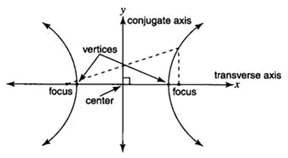Hyperbolas

A hyperbola is the set of all points in a plane such that the absolute value of the difference of the distances between two fixed points stays constant. The two given points are the foci of the hyperbola, and the midpoint of the segment joining the foci is the center of the hyperbola. The hyperbola looks like two opposing "U-shaped" curves.A hyperbola has two axes of symmetry. The axis along the direction the hyperbola opens is called the transverse axis. The conjugate axis passes through the center of the hyperbola and is perpendicular to the transverse axis. The points of intersection of the hyperbola and the transverse axis are called the vertices of the hyperbola.